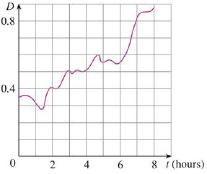Chapter 5.4, Problem 72E### Single Variable Calculus: Early Tr...

8th Edition
James Stewart
ISBN: 9781305270343

#### Solutions

Chapter
Section### Single Variable Calculus: Early Tr...

8th Edition
James Stewart
ISBN: 9781305270343
Textbook Problem

# Shown is the graph of traffic on an Internet service provider’s T1 data line from midnight to 8:00 am. D is the data throughput, measured in megabits per second. Use the Midpoint Rule to estimate the total amount of data transmitted during that time period.To determine

To calculate: The total amount of data transmitted during 8 hours’ time period.

Explanation

Given information:

Duration of data transmission is t=0 to t=8hours.

The general expression for midpoint rule is shown below:

abf(x)dxi=1nf(x¯i)Δx=Δx(f(x¯1)+....+f(x¯n))

Apply the conditions for midpoint rule as follows:

Δx=ban (1)

x¯i=12(xi1+xi) (2)

Here, width of the subinterval is Δx, the upper limit is b, the lower limit is a, the number of subinterval is n, and the midpoint of (xi1,xi) is x¯i.

Calculation:

Find the width of the subinterval (Δt) using Equation (1).

Consider the number of subintervals as 4.

Substitute 4 for n, 8 hours for b and 0 for a in Equation (1).

Δt=804=2hours

The expression to find the total amount of data transmitted [D(8)D(0)] during the time t=0 to t=8hours using midpoint rule as shown below.

[D(8)D(0)]=08D(t)dtΔt(D(t¯1)+D(t¯2)+D(t¯3)+D(t¯4)) (3)

Here, the amount of data transmitted at time t¯1 is D(

### Still sussing out bartleby?

Check out a sample textbook solution.

See a sample solution

#### The Solution to Your Study Problems

Bartleby provides explanations to thousands of textbook problems written by our experts, many with advanced degrees!

Get Started

#### Find more solutions based on key concepts